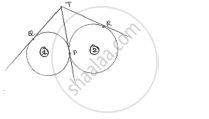Share

# Two Circles Touch Externally at a Point P. from a Point T on the Tangent at P, Tangents Tq and Tr Are Drawn to the Circles with Points of Contact Q and E Respectively. Prove that Tq = Tr. - CBSE Class 10 - Mathematics

ConceptCircles Examples and Solutions

#### Question

Two circles touch externally at a point P. from a point T on the tangent at P, tangents TQ and TR are drawn to the circles with points of contact Q and E respectively. Prove that TQ = TR.

#### SolutionLet the circles be represented by (i) & (ii) respectively

TQ, TP are tangents to (i)

TP, TR are tangents to (ii)

We know that

The tangents drawn from external point to the circle will be equal in length.

For circle (i), TQ = TP …. (i)

For circle (ii), TP = TR …. (ii)

From (i) & (ii) TQ = TR

Is there an error in this question or solution?

#### Video TutorialsVIEW ALL 

Solution Two Circles Touch Externally at a Point P. from a Point T on the Tangent at P, Tangents Tq and Tr Are Drawn to the Circles with Points of Contact Q and E Respectively. Prove that Tq = Tr. Concept: Circles Examples and Solutions.
S